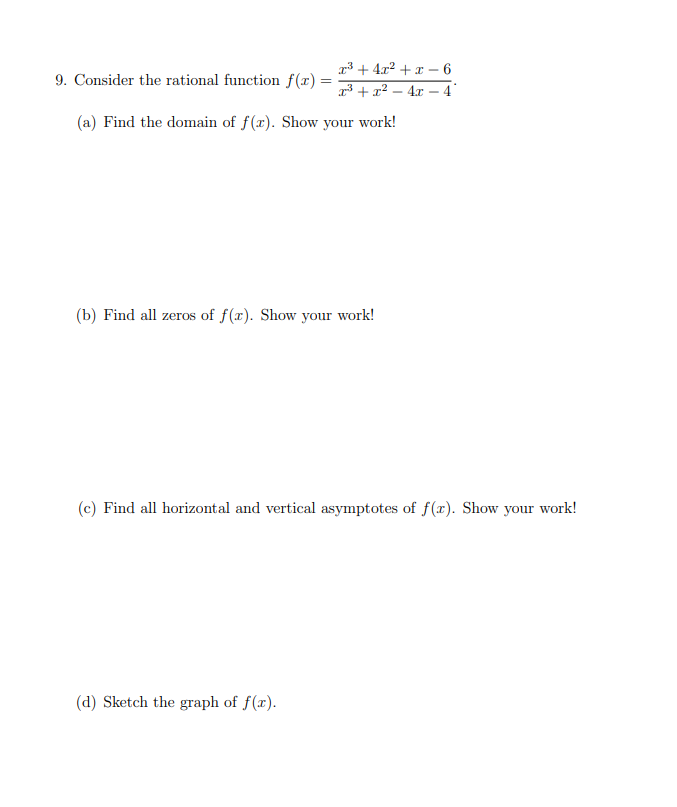# r342 - 69. Consider the rational function f(x)= 34 -4(a) Find the domain of f(x). Show your work!(b) Find all zeros of f(x). Show your work!(c) Find all horizontal and vertical asymptotes of f(x). Show your work!(d) Sketch the graph of f(x)

Question
1 views

I need help with question 9.a only you can ingnore that other parts of the question.help_outlineImage Transcriptioncloser342 - 6 9. Consider the rational function f(x)= 34 -4 (a) Find the domain of f(x). Show your work! (b) Find all zeros of f(x). Show your work! (c) Find all horizontal and vertical asymptotes of f(x). Show your work! (d) Sketch the graph of f(x) fullscreen
check_circle

Step 1

To find the domain of the given function f(x).

Step 2

Given information:

The function is given as,

Step 3

Domain is the set of input or argument values for which the function is r...

### Want to see the full answer?

See Solution

#### Want to see this answer and more?

Solutions are written by subject experts who are available 24/7. Questions are typically answered within 1 hour.*

See Solution
*Response times may vary by subject and question.
Tagged in

### Algebra